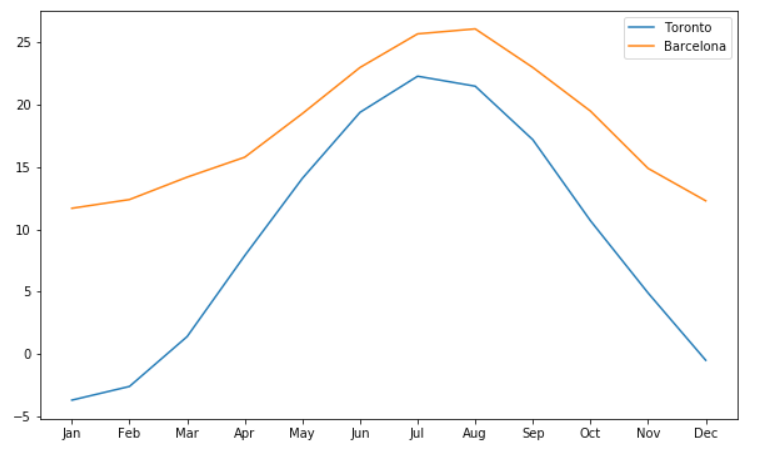Deals Of The Week - hours only!Up to 80% off on all courses and bundles.-Close
Introduction
Line plots
6. Axis tick labels
Multiple histograms
Other plot types
Summary

## Instruction

Great! Now, we have another problem: on the x-axis, instead of month names from our DataFrame (Jan, Feb, Mar…), we can only see numbers from 0 to 12. To change that, we need to add the following line of code somewhere before plt.show():

plt.xticks(range(len(climate['Month'])), climate['Month'])

plt.xticks takes two arguments. The first argument is the list of positions at which ticks (month names) should be placed. We use range() – the same function we used when creating loops – to create a range of numbers from zero to the length of climate['Month'] column, which is 12.

The second argument is a list of labels that should be placed at the positions specified by the first argument. In this case, we want to use the values in the climate['Month'] column.

The result looks like this:## Exercise

Use the values of the year column from the books DataFrame as the labels for the x-axis ticks.

### Stuck? Here's a hint!

Use the following code:

plt.xticks(range(len(books['year'])), books['year'])